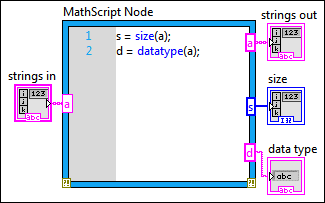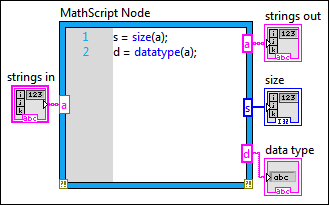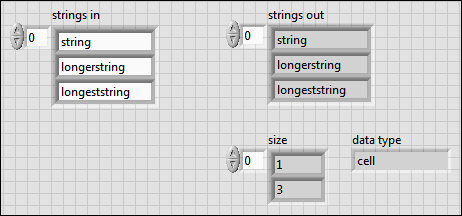# Passing Data in and out of the MathScript Node (MathScript RT Module)

LabVIEW 2012 MathScript RT Module Help

Edition Date: June 2012

Part Number: 373123C-01

»View Product InfoDownload Help (Windows Only)

If you pass a cluster or a 1D array of strings into the MathScript Node, the node accepts those data types as different, corresponding data types in the MathScript environment.

## Passing Clusters into a MathScript Node

You can pass LabVIEW clusters into a MathScript Node. If the following conditions are true, a cluster becomes a structure in the MathScript environment with fields that have the same names and values as the names and values of the individual elements of the cluster:

• You must label individual elements of the cluster. Labels must start with an alphabetic character and can contain only alphanumeric characters and underscores without any spaces.Note  You can use the function is_validvarname to validate cluster element labels.
• Use the following data types for individual elements of the cluster:
• Scalar, 1D array, or 2D array of the following data types:
• Boolean
• Numeric
• (Control Design and Simulation Module) State-space model, zero-pole-gain model, or transfer function model
• Scalar or 1D array of strings
• Real matrix
• Complex matrix
• Cluster that meets the conditions for individual elements of a cluster

If you do not follow these conditions, the wire to the input of the MathScript Node breaks.

## Passing Structures out of a MathScript Node

If you pass a structure out of a MathScript Node, the structure becomes a cluster in the LabVIEW environment. You can pass a structure under the condition that you use the following data types for individual elements of the structure:

• Scalar, 1D array, or 2D array of the following data types:
• Boolean
• Numeric
• (Control Design and Simulation Module) State-space model, zero-pole-gain model, or transfer function model
• 1D or 2D array of strings
• Structure that meets the conditions for individual elements of a structure

If you do not follow these conditions, the output terminal to the MathScript Node breaks.

### Avoiding Different Data Types in Corresponding Fields of a Structure Array

When passing structures out of a MathScript Node, you cannot use different data types in corresponding fields of a structure array or the output terminal to the node breaks. The following example shows different data types in fields of a two-element structure array:

a(1).b = 5;

a(2).b = 'string';

In structure a(1), the data type of field b is integer. In structure a(2), the data type of field b is string. LabVIEW does not coerce an integer to a string, so the structure does not pass out of the node.

## Passing Arrays of Strings into a MathScript Node

When you pass a 1D array of strings from the block diagram into a MathScript Node, by default, the array of strings becomes a 2D padded array in the MathScript environment. The following example shows how the MathScript Node accepts a LabVIEW array of strings with different lengths as a 2D padded array.Note  The previous front panel shows the strings out indicator configured with the '\' Codes Display display type, which displays backslash codes for all non-displayable characters.

In this example, the LabVIEW array of strings becomes a padded 2D array of characters with 3 rows and 13 columns. MathScript pads the first two rows with spaces until those rows equal the length of the longest string. If you pass the padded 2D array out of the MathScript Node, it becomes a 1D array of strings in the LabVIEW environment; however, it retains the spaces that MathScript applies.

### Passing an Array of Strings as a Cell Array

You also can pass a 1D array of strings into the MathScript Node and specify that each string maintains its own length. Right-click the input terminal on the MathScript Node and select String Representation»Cell Array from the shortcut menu.

The following example shows how the MathScript Node accepts a 1D array of strings with different lengths when you specify the string representation as a cell array.When you pass this LabVIEW array of strings into the MathScript environment, it becomes a cell array with 1 row and 3 columns, and each string maintains its own length. If you pass this cell array out of the node, the cell array becomes a 1D array of strings in the LabVIEW environment.

Not Helpful